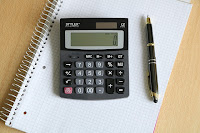## Saturday, February 6, 2021

### Batch Size Calculations|Pharma Calculations

Batch size calculations are very basic and daily used calculations which are regularly performed by the professionals working in the pharmaceutical industries.

The purpose of these calculations is to estimate quick values when one or two values are given and another is to determine without any documentation.

It is the fact that usually all values are given in Batch Manufacturing Record (BMR) but these simple calculations will help us to calculate all required values when we have no BMR.1. Batch Size Calculation

(In Kilograms)

1. Batch Size Calculation

(In Numbers)

1. Tablet Weight Calculation

1. Basic API Calculation

1. Batch Size Calculation

(In Kilograms)

Batch size in kilograms is the total standard weight of a final product in kilograms.

Example

• Suppose we have a tablet product having 200,000 tablets. Weight of an individual tablet is 300 mg.

What will be the total batch size of the product in kilograms?

Solution

It's simple unit rule calculation and the  solution is as follow,

As we know

Weight of 1 tablet is = 300 mg

To calculate the weight of 200,000 tablets simply multiply 300 mg to the batch size of the product in the number of tablets which is 200,000.

So

300 mg×200,000=60,000,000 mg

Convert it in kilograms

So First Convert milligrams into grams as follow

As we know 1gram=1000 mg so divide the above value to 1000 to get value in grams

60,000,000 mg / 1000=60,000 gram

Now convert grams into kilograms

As we know 1 kg =1000 gm so divide

above value with 1000 to get kilograms

as follow

60,000 gm/ 1000= 60 kg

Conclusion

• The Required standard batch size of our product in kilograms is 60 kg

Hint

• A simple rule to remember for weight conversion calculations is that when we have to convert Higher values to Lower values (Kg to mg) then use the multiplication rule.

• Multiply kilograms to 1000 to get value in the gram and then again multiply by 1000 to get values in milligrams(kg×1000×1000).

• When we have to convert lower values to Higher values (mg to kg) then use the Division rule.

• Divide milligrams to 1000 to get value in the gram and then again Divide by 1000 to get values in kilograms(mg÷1000÷1000).

Reconciliation in pharmaceutical industries.

1. Batch Size Calculation

(In Numbers)

Batch size in Numbers is the total number of units of a final product.

Example

• Suppose we have a tablet product having 60-kilograms weight and Weight of individual tablets is 200 mg.

What will be the total batch size of the product in Numbers?

Solution

It is also a simple unit rule calculation and solution is as follows.

As we know

Weight of 1 tablet is = 200 mg

To calculate the number of total tablets produced from 60 kg use following

Batch Size (In Number) = Batch Size in Milligrams ÷ Weight Of Tablet

So

First of all, convert 60 kilograms into milligrams as follow

60 kg×1000×1000=60,000,000 mg

Divide the value of milligrams by the weight of an individual tablet which is 200 mg in this case.

Or

Put values in the above formula as

60,000,0000/200 mg = 300,000 Tablets

Conclusion

• The Required Standard batch size of our product in terms of numbers is 300,000 Tablets.

HVAC or Heating Ventilation & Airconditioning System.

1. Tablet Weight calculation

This calculation is helpful when we have numbers of tablets and standard weight of the product in kilograms but we do not have weight of the tablet and we want to calculate the individual tablet weight.

Example

• Suppose we have a tablet product having 80-kilograms standard weight and the total batch size in terms of numbers is 200,000 Tablets.

What will be the weight of the individual tablet?

Solution

As we know

The standard weight of our product is 80 kilograms and the number of tablets is 200,000 Tablets.

To calculate the weight of individual tablet use following formula

Weight of Tab=Batch Size in mg ÷ Batch Size in numbers

So first we will convert kilograms into milligrams and then we will divide it with the number of tablets as

Weight of Tab= 80 kg ×1000×1000 / 200,000

Weight of Tablet = 400 mg

Conclusion

• The Weight of an individual tablet is

400 mg

Calculation of compression machine capacity.

1. Basic API Calculation

This basic calculation helps us to calculate the basic amount of API (Active material) required for specific batch size.

Example

• Calculate the amount of Metformin HCl required for a tablet formulation having 200,000 tablets and tablet strength is 500 mg/ tablet.

Solution

As we know

A single tablet contains 500 mg Metformin HCl so to calculate Metformin HCl for 200,000 tablets we will use the following formula

Amount of API=Tablet Strength × Batch Size in numbers

For the above example put values in formula as follow

Amount of API = 500 mg × 200,000 =100,000,000 mg

For kilograms divide it by 1000 × 1000 as follow

100,000,000 /1000×1000 = 100 kg

Conclusion

• Amount of Metformin HCl required for 200,000 tablets having 500 mg potency is 100 kg.

Note

• Its basic API calculation.

• For salt/factor removal or moisture content compensation read following details

Popular Posts:

Easy Steps to calculate Double cone blender capacity.

Line Clearance in pharmaceuticals.

GMP vs cGMP and 21 CFR.

Qualification|FAT|SAT|DQ|IQ|OQ|PQ

Difference between Validation & Calibration.

100 MCQs to revise your pharmaceutical knowledge.

1.2.